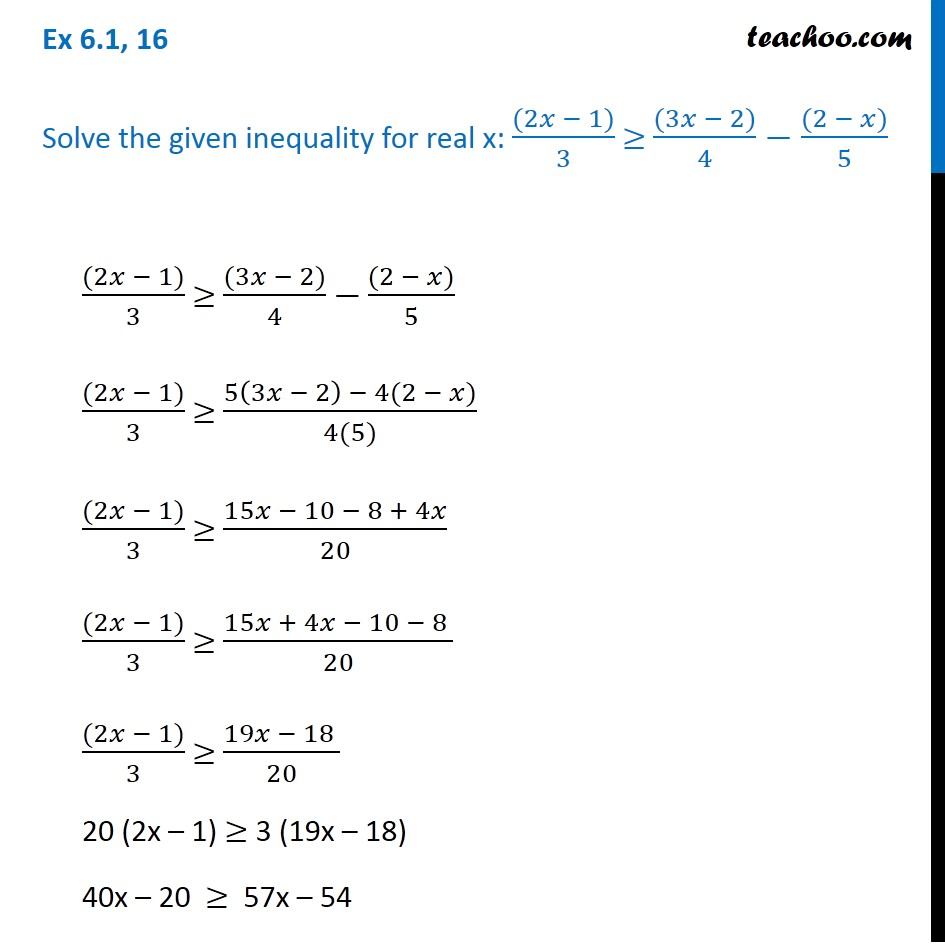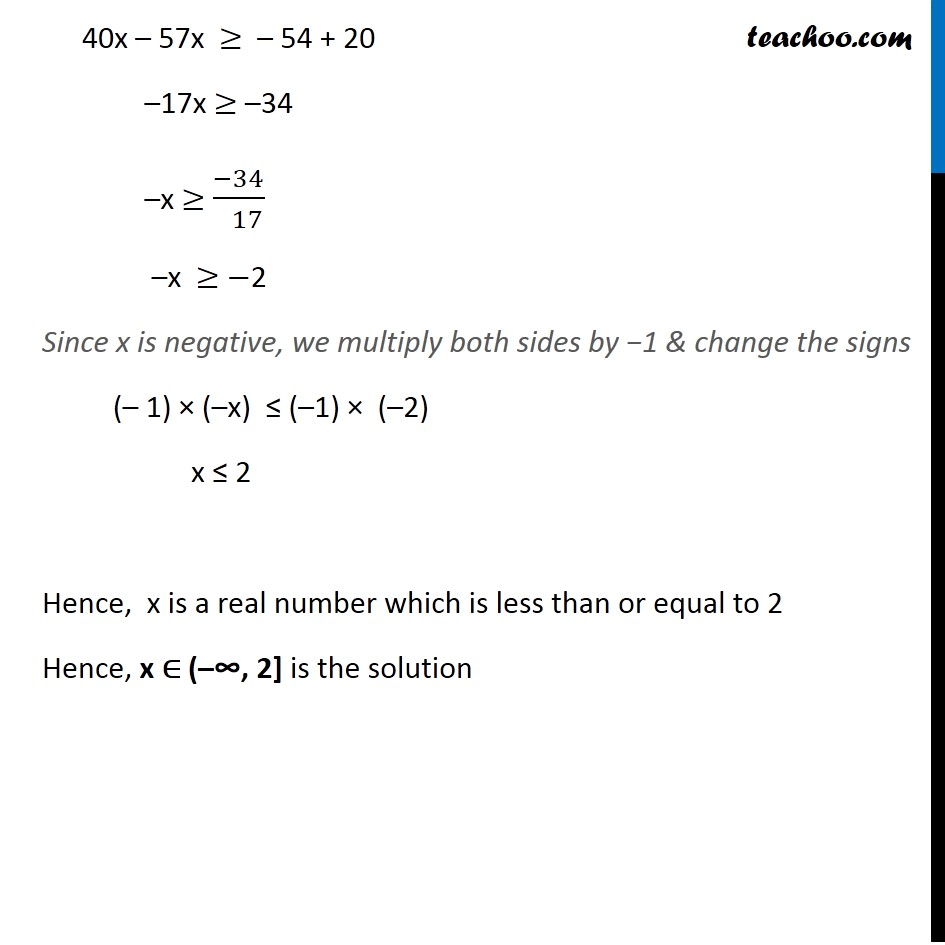Ex 6.1

Chapter 6 Class 11 Linear Inequalities (Term 2)
Serial order wise641 students have Teachoo Black. What are you waiting for?

### Transcript

Ex 6.1, 16 Solve the given inequality for real x: ((2𝑥 − 1))/3 ≥ ((3𝑥 − 2))/4−((2 − 𝑥))/5 ((2𝑥 − 1))/3 ≥ ((3𝑥 − 2))/4 −((2 − 𝑥))/5 ((2𝑥 − 1))/3 ≥ (5(3𝑥 − 2) − 4(2 − 𝑥))/(4(5)) ((2𝑥 − 1))/3 ≥ (15𝑥 − 10 − 8 + 4𝑥)/20 ((2𝑥 − 1))/3 ≥ (15𝑥 + 4𝑥 − 10 − 8 )/20 ((2𝑥 − 1))/3 ≥ (19𝑥 − 18 )/20 20 (2x – 1) ≥ 3 (19x – 18) 40x – 20 ≥ 57x – 54 40x – 57x ≥ – 54 + 20 –17x ≥ –34 –x ≥ (−34)/( 17) –x ≥ −2 Since x is negative, we multiply both sides by −1 & change the signs (– 1) × (–x) ≤ (–1) × (–2) x ≤ 2 Hence, x is a real number which is less than or equal to 2 Hence, x ∈ (–∞, 2] is the solution